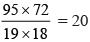Courses

# High Order Thinking Skills -1

## 20 Questions MCQ Test Mathematics Olympiad Class 7 | High Order Thinking Skills -1

Description
This mock test of High Order Thinking Skills -1 for Class 7 helps you for every Class 7 entrance exam. This contains 20 Multiple Choice Questions for Class 7 High Order Thinking Skills -1 (mcq) to study with solutions a complete question bank. The solved questions answers in this High Order Thinking Skills -1 quiz give you a good mix of easy questions and tough questions. Class 7 students definitely take this High Order Thinking Skills -1 exercise for a better result in the exam. You can find other High Order Thinking Skills -1 extra questions, long questions & short questions for Class 7 on EduRev as well by searching above.
QUESTION: 1

### What is the smallest number which when divided by 16, 36 & 80 leaves a remainder 7 in each case?

Solution:

LCM of 16, 36, 80 = 720 Required smallest number = 720 + 7 = 727

QUESTION: 2

### Which of the following cannot be the sides of any triangle?

Solution:

2 + 12 < 15,
So, 2, 12, 15 cannot be sides of a triangle.

QUESTION: 3

### If Raju runs a distance of 7 km 800m in going round a rectangular stadium ground three times then what is the length of the ground if its width is 330m?

Solution:

Distance = 7 km 800 m = 7800 m Perimeter × 3 = 7800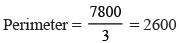2 (x + 330) = 2600
⇒ x + 330 = 1300
⇒ x = 1300 – 330 = 970m

QUESTION: 4

What is the number of square tiles of size 15cm required for covering the floor of a room having dimension 9m × 6m?

Solution:

Length of rectangular floor of room= 9m

breadth of rectangular floor= 6m

∴Area of rectangular floor = length* breadth

=   9*6

= 54 m²

Side of Square tile= 15cm

Now we need to convert Side of Square tile in meters (since unit of all quantity has to be same for ease of calculation)= 15/100=0.15m

Area of square tile = side²

= 0.15²

=0.0225 m²

Now number of tiles required to cover the floor =(area of floor of room)/(area of 1 tile)

= (54)/(0.0225)

=2400 tiles

QUESTION: 5

If Rs. 7140 is divided among A, B, C in the ratio 2 : 3 : 5. What is the share of B?

Solution:

2x + 3x + 5x = 7140
⇒ 10x = 7140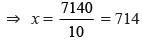∵ Share of B = 714 × 3 = 2142

QUESTION: 6

A wire of length 172cm is bent in the form of a rectangle such that its length is 7cm more than its breadth. What is its breadth?

Solution:

Let the breadth be b cm
Length = (b + 7) cm
Perimeter = 2 (l + b)
= 2 (b + 7 + b) = 2 (2b + 7)
⇒ 2 (2b + 7) = 172
⇒ 2b + 7 = 86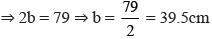QUESTION: 7

The sum of three consecutive even numbers be 222. Which of them is largest even number?

Solution:

Let three consecutive even numbers be x, x + 2, x + 4.
∴ x + x + 2 + x + 4 = 222
⇒ 3x + 6 = 222
⇒ 3x = 222 – 6
⇒ 3x = 216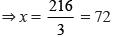Largest even number = x + 4
= 72 + 4 = 76

QUESTION: 8

26 men can dig a hole in 12 days. How many men can dig a hole in 4 days?

Solution:

∵ In 12 days a hole can be dig by 26 men
∴ In 1 day a hole can be dig by 26 × 12
∴ In 4 days a hole can be dig by =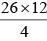= 78 men

QUESTION: 9

In covering 121km a car consumes 11 litre of petrol. How many kilometers will it go in 15 litre of petrol?

Solution:

∵ In 11 litres a car covers 121 km.
∵ In 1 litre a car covers 121/11 = 11 km.
∴ In 15 litres a car covers 11 × 15 = 165 km.

QUESTION: 10

The cost of 5 bars of a soap is Rs. 92.50. What is the cost of three dozen bars of soap?

Solution:

∴ The cost of 5 bars of soap = Rs. 92.50
∴ The cost of 1 bar of soap =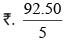∴ The cost of 36 bars of soap =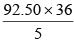= 666

QUESTION: 11

The sides of a triangle are in the ratio 1 : 3 : 5 and its perimeter is 90cm. What is the length of smallest side?

Solution:

x + 3x + 5x = 90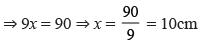∴ Length of smallest side = x = 10cm

QUESTION: 12

In a fort 550 men had provisions for 56 days. How many days will it last for 700 men?

Solution:

∵ 550 men had provision for 56 days.
∴ 1 man had provision for 56 × 550
∴ 700 men had provision for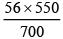= 44 days

QUESTION: 13

The cost of fencing a square field at Rs. 125 per metre is Rs. 16000. What is the area of that field?

Solution:

Perimeter of square =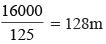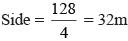Area = (32)2 = 1024 m2

QUESTION: 14

The distance between Raman’s house and his office is 18km. He covers 8km 65m by scooter, 4km 325m by bus and rest on feet. How much distance does he covers by walking?

Solution:

Distance covered by walking  18km – [8 km 65 m + 4 km 325 m] 18km – 12km 390m = 5km 610m

QUESTION: 15

What is simplified value of​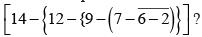Solution:

[14 – {12 – {9 – (7 – 6 −2 )}]
= [14 – {12 – {9 – (7 – 4)}]
= [14 – {12 – {9 – 3}]
= [14 – {12 – 6}]
= 14 – 6 = 8

QUESTION: 16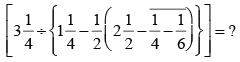Solution: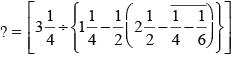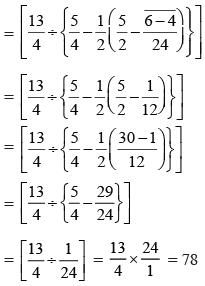QUESTION: 17

An electronic device makes a beep after every 12 minutes. Another device makes a beep after every 18 minutes. They beeped together at 6 a.m. At what time they next beep together?

Solution:

LCM of 12 and 18 = 36 minutes. 6 a.m. & 36 minutes = 6 : 36

QUESTION: 18

What is the least number which is divisible by 3, 7, 9, 12, 15 & 18 ?

Solution: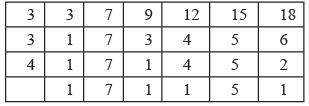∴ 3 × 3 × 4 × 7 × 5
= 420 × 3 = 1260
LCM of 3, 7, 9, 12, 15, 18 = 1260

QUESTION: 19

The area of a rectangular carpet is 120m2 and its perimeter is 46m. What is the length of the diagonal?

Solution:

2 (l + b) = 46 ⇒l + b = 23 ……..(1)
lb = 120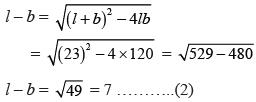From (1) & (2) 2l = 30 ⇒l = 15
b = 8
∴ Length of diagonal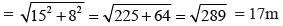QUESTION: 20

How many envelops can be made out of a sheet of paper 95 cm by 72 cm. If each envelops requires a paper of size 19 cm to 18 cm?

Solution:

Number of envelops =